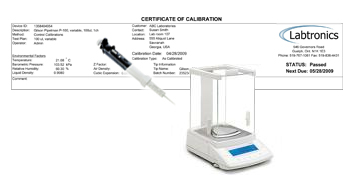## Tuesday, December 25, 2012

### Pipette calibration : Procedure and Calculations

Principle:
Under a constant temperature and atmospheric pressure, the density of distilled water is constant. The volume of water can be determined by weighting dispensed water. The calibration of pipette is carried out by gravimetric method. When determining the volume of water, the accuracy of measurements is effected by ambient temperature, atmospheric pressure and relative humidity. These factors are usually combined to give the Z factor, used in calculation of volume of water. Then the calculated volume of water is compared with the theoretical volume to determine the accuracy and precision of the pipette.

A Micro Pipette

Material and equipment:
• Ppipette and tips
• 50 ml beaker and plastic medicine cup
• Distilled water
• Temperature meter ( ±0.1℃ )
• Analytical balance ( ±1.0 mg )
• Atmospheric pressure meterPipette Calibration
Procedure:
• Determine the water temperature and record it.
• Place a beaker filled with distilled water into analytic balance and close the door of balance waiting for equilibrium of inner vapor.
• Place a plastic medicine cup on the pan and adjust the weight to zero.Put a tip onto the pipette and set the volume which is to be tested.
• Pre-rinse the tip: aspirate and dispense the setting volume three times and press the push – button on the second stop to remove any remaining liquid.
• Press the push-button to the first positive stop. Hold the pipette vertically, immerse the tip so that 1-4 mm in the liquid and release the push button slowly and smoothly to aspirate the liquid.
• Wait one second and withdraw the tip from the liquid.
• Wipe any droplets away from the outside of the tip using a kimwipe.
• Place the end of the tip against the inside wall of the plastic cup at an angle of 10-40 °.
• Press the push-button smoothly to the first stop. Wait one second, change new site and press the push-button on the second stop.
• Keeping the push-button press to the end, remove the pipette by drawing the tip along the inside surface of the plastic cup and release the push button.
• Close the door of balance and record the value on the balance display after it has stabilized.
• Repeat step (6) to (12) 14 times ( the weight display of balance should be adjusted to zero every time)
•  Eject the tip.
• Pipette should be calibrated every three months routinely.
Factors Affecting the Accuracy of Air Displacement Pipettes
• Temperature
Temperature has many effects on pipetting accuracy. The factor that has the greatest effect
is the temperature difference between the used delivery device and liquid. The air gap (dead
air volume) between the liquid surface and the piston experiences thermal expansion effects
according to the case. This either reduces or increases the liquid amount aspirated into the tip
along with other effects.
• Density
The density (mass/volume ratio) affects the liquid volume that is aspirated into the tip. A smaller dose of liquid with higher density than water is aspirated compared to similar operation
with water. With lower density liquids the effect is the opposite. This is caused by the flexible
dead air volume along with the earth gravity. The density of liquids varies also according to the
temperature. Typically the density for water is 0.998 kg/dm3, for ethanol 0.79 kg/ dm3 and for sulfuric acid (95-98% H 2SO4) 1.84 kg/dm3 (the values apply at temperature of 0 °C).
• Altitude
The geographic altitude affects the accuracy through air pressure.  The air pressure decreases
in higher altitudes and the conversion factor Z decreases as well.  Also, with some liquids the
boiling point decreases quite close to room temperature, which will increase the evaporation
loss dramatically.
• Pipetting position
Pipetting position can also lead to inaccuracy.

Calculation:

Conversion of mass to volume

V = (w + e) x Z

V = Volume (µl)  w = Weight (mg)
e = Evaporation loss (mg)
Z = Conversion factor for mg/µl conversion

Accuracy (systematic error)

Accuracy is the difference between the dispensed volume and the selected volume of a pipette.
A = V -V0

A = Accuracy
V = Mean volume
V0 = Nominal volume

Accuracy can be expressed as a relative value:
A% =( 100% x A) / V0

Convert the weight unit of measured value into the volume unit of measured value using the following formula:

Volume ( ml or ul ) = Weight ( mg or ug ) x Z

Z value : conversion factor, which is conversion of density .

Mean Weight = (sample replicate 1 + sample replicate 2 + (etc.))/ Number of Replicates

Mean Volume (Corrected Mean) = Mean Weight × ZFactor

% Inaccuracy = [(Corrected Mean – True Value) ÷ True Value] × 100

Standard deviation can be expressed as a relative value as cv.

% CV (Coefficient of Variation) = (Standard Deviation / Corrected Mean) × 100

Calculate the average ( Mean ), accuracy, standard deviation (S.D.) and imprecision ( C.V. ) using the
following formula:

n 　　　　　　　　　 　　　n 　　－ 2

Σ Xi 　　　　　　　 　　　Σ (Xi-X)

－　　 i=1 　　　　　　　　　　　 i=1 　　　　　1 / 2

(Mean) X ═ ---------------- ; 　　S.D. ═（----------------- ）

N 　　　　　 　　　　　　　N

N: number of measured value

S.D. 　　　　　　　　　　　Measured value

C.V. = ----------- X 100％ ； Accuracy = ------------------- X 100％

Mean 　　　　　　　　　　　Theoretical value

• Accuracy value must be 99-101 % and C.V. value must be less than 1 %.
References
• Y.C.Chang (Apr. 14, 2007), Laboratory manual of  Clinical  Biochem.  Res.  Lab.                                                                    Dept. of Res. & Edu.Taipei Veterans General Hospital.
• Roger Schultz, Wisconsin State Laboratory of Hygiene, December 5, 2007
• Thermo Pipetting Guide